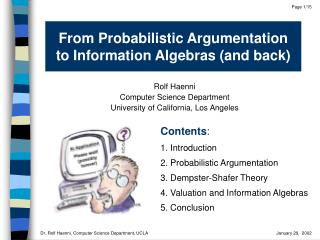DownloadDownload PresentationFrom Probabilistic Argumentation to Information Algebras (and back)

From Probabilistic Argumentation to Information Algebras (and back)

Télécharger la présentationFrom Probabilistic Argumentation to Information Algebras (and back)

- - - - - - - - - - - - - - - - - - - - - - - - - - - E N D - - - - - - - - - - - - - - - - - - - - - - - - - - -
Presentation Transcript

1. From Probabilistic Argumentation to Information Algebras (and back) Rolf Haenni Computer Science DepartmentUniversity of California, Los Angeles Contents: 1. Introduction 2. Probabilistic Argumentation 3. Dempster-Shafer Theory 4. Valuation and Information Algebras 5. Conclusion

2. 1) elaborate possible answers or alternatives 2) list pros and cons (for each answer or alternative) 3) measure or weigh pros and cons (for each answer or alternative) 4) acceptanswer or choose alternative with the maximal total weight (or gather more information, if necessary) Cons Pros 1. Introduction Reasoning and deciding under uncertainty is common in everyone’s daily life:

3. Example: who qualifies for the play-offs?

4. The most popular formal approach is different: 1) elaborate possible answers or alternatives 2) build probabilistic model (usually a Bayesian network) 3) compute posterior probabilities (for each answer or alternative) 4) apply decision theory (maximize expected utility or minimize expected cost)  this disrespects: 1) the true nature of uncertain reasoning observed in everyone’s daily life 2) the existence of partial or total ignorance  e.g. knowing that p(head)=0.5 is different than not knowing the probability p(head)

5. 1. Modeling Uncertainknowledge Knowledge R a®R Fact: a® (R ® S) R ® S Simple rule: a®  General rule: More general: Ingredients: - possible states - risk elements - interpretations - unknown circumst. - uncertain outcomes - measurement errors • propositions • assumptions • prop. formulas 2. Probabilistic Argumentation

6. 2. Qualitative Analysis arguments pro/contra Hypothesis hypothesis  open question about unknownor future world a) arguments in favor of  combination of assumptions proving the hypothesis b) counter-arguments against  combination of assumptions disproving the hypothesis Example: a1®X a4 a1 a2 arguments (a2a3) ®Y hypothesis knowledgebase a1a3 (XY) ®Z Z a2 a5 a4®Z counter-arguments a3 a5 (a5Y) ®Z

7. Remarks: 1) 2) support is sub-additive: 3) possibility is super-additive: 4) support and possibility are non-monotone! 5) and means total ignorance 3. Quantitative Analysis a) define probability distributionover  e.g. independent probabilities for each assumption b) compute degree of support:  conditional probability that at least one argument is true (given no conflicts) c) compute degree of possibility:  one minus conditional probability that at least one counter-argument is true (given no conflicts)

8. Y Additive: H E X Sub-additive: Z H Y E X J. Bernoulli: “Ars Conjectandi”, 1713 3. Dempster-Shafer Theory

9. 1. Modeling a) define variables, domains, and frames with b) define mass functions with  knowledge base: 2. Quantitative Analysis: (sub-additive)  belief:  plausibility: (super-additive)

10. and Degree ofSupport Arguments Belief Probabilistic ArgumentationSystem Dempster-Shafer MassFunctions Hypothesis Hypothesis Degree ofPossibility Counter-Arguments Plausibility Remark:  Every probabilistic argumentation system can be transformed into a set of mass function such that for all .

11. a1 a1®X X (a2a3) ®Y (XY) ®Z a2 a4 a4®Z Y Z a3 (a5Y) ®Z a5 4. Valuation and Information Algebras • Knowledge is usually composed of small pieces of information • Each piece of information affects only a few variables

12. set of variables • valuation  “piece of information” Framework: • domain •  set of valuations with •  all valuations 1988: Local Computation (for probabilistic inference), Lauritzen & Spiegelhalter, J. Royal Statistical Society 1990: Valuations, Axioms, Propagation in Join TreesShenoy & Shafer, UAI’90 & Readings in Uncertain Reas. 2000: Valuation & Information Algebras, Shenoy & Kohlas, Handbook of Defeasible Reas. & Management of Uncert. 2002: Ordered Valuation Algebras, Resource-BoundedApproximation, Haenni, AI Journal (?)

13. Knowledge base: Operations: • combination:  (aggregated information) • marginalization:  (information focussed to ) Problem of Inference: • compute  marginalize the joint valuation to the domain of interest

14. Axioms:

15. Valuation algebra:  system satisfying (A1) to (A6) • probability potentials (CPT’s from Bayesian network) • belief potentials (in the sense of Dempster-Shafer) • possibility functions Information algebra:  system satisfying (A1) to (A7) • propositional logic • constraint systems • systems of linear equations and inequalities • relational algebra • probabilistic argumentation systems

16. 5. Conclusion • Probabilistic argumentation is a natural approach to reasoning under uncertainty. • From a quantitative point of view, probabilistic argumentation is equivalent to Dempster-Shafer theory. • The abstract framework of valuation and information algebras is a powerful tool that allows to better under-stand the nature of information and its computational challenges. • Convenient resource-bounded or anytime algorithms have been developed for probabilistic argumentation, Dempster-Shafer theory, and valuation algebras.